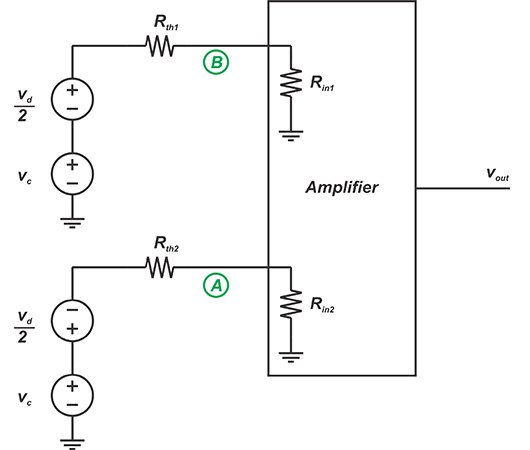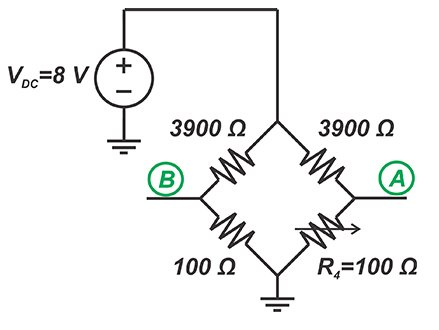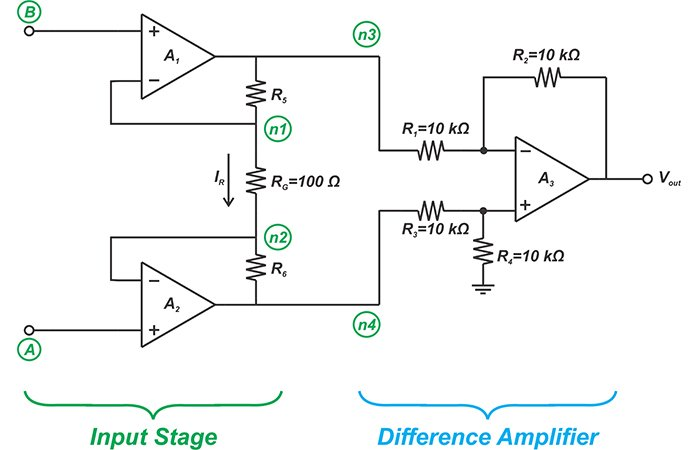In a previous article, we discussed that instrumentation amplifiers (in-amps) should have a high common-mode rejection to successfully extract a small differential signal. In this article, we’ll see that in addition to having high common-mode rejection, in-amps should provide high and equal input impedances. Besides, we’ll look at the advantages and disadvantages of a commonly-used in-amp often referred to as the three-op amp in-amp.

### An In-Amp Should Provide High, Balanced Impedance at Its Inputs

Let’s examine the bridge measurement system depicted below to discuss why an in-amp should provide high and equal input impedances.##### Figure 1

The bridge and amplifier can be modeled by the following Thevenin equivalent.##### Figure 2

Here, Rth1 and Rth2 are the equivalent resistances of the two bridge branches. Besides, the voltages at nodes A and B in Figure 1 are decomposed into differential (vd) and common-mode (vc) components given by:

$v_c=\frac {v_A+v_B}{2}$

$v_d=v_A-v_B$

Assume that the bridge is balanced and the resistor values are as shown in the following figure.##### Figure 3

In this case, the values of the Thevenin equivalent circuit can be found as: vd=0, vc=200 mV, and Rth1=Rth2=97.5 Ω. Moreover, suppose that the following difference amplifier is used as the bridge amplifier.##### Figure 4

In this case, the amplifier input impedance at nodes A and B will be respectively 200 kΩ and 100 kΩ. Hence, considering the equivalent model in Figure 2, we have:

$v_A= \frac{R_{in2}}{R_{in2}+R_{th2}}v_c=\frac{200 k\Omega}{200 k\Omega + 97.5\Omega} \times 200 mV=199.90 mV$
$v_B= \frac{R_{in1}}{R_{in1}+R_{th1}}v_c=\frac{100 k\Omega}{100 k\Omega + 97.5\Omega} \times 200 mV=199.81 mV$

Since the two inputs of the amplifier exhibit different impedances to ground, the common-mode voltage of the bridge produces unequal signals at the amplifier inputs. Assuming that the amplifier can completely reject any common-mode signals, i.e. Acm=0, we can find the output voltage as:

$v_{out}=A_d \times (v_A-v_B)=1 \times (199.90-199.81)=90 \mu V$

While the amplifier was assumed to have infinite common-mode rejection ratio (CMRR), the unbalanced loading effect of the amplifier allows the common-mode voltage to produce an error signal at the output. This effect will be even more serious if we use an amplifier with lower input impedances. Hence, it is best for the amplifier input terminals to have high and equal impedances.

### The Classic Three-Op amp In-Amp

A commonly used in-amp circuit that provides a high CMRR along with balanced, high input impedances is the three-op amp structure depicted in Figure 5.##### Figure 5

The above schematic can be decomposed into two sections. The input stage acts mainly as a buffer. The output stage is a difference amplifier that was discussed in the previous article. As we’ll see below, the three-op amp in-amp employs a clever architecture with several interesting features.

### High, Balanced Input Impedance

Both nodes A and B are connected to the non-inverting input of the corresponding op amps. This gives high, balanced input impedances without resorting to complicated feedback techniques.

### The Input Stage Can Provide High Differential Gain and CMRR

Let’s first calculate the differential gain of the input stage. The negative feedback along with the high gain of the op amps will force both the inverting and non-inverting inputs of A1 and A2 to have the same voltage. Hence, we have:

$v_{n1}=v_B$

$v_{n2}=v_A$

The current through RG, and hence through R5 and R6, will be:

$I_R=\frac{v_{n1}-v_{n2}}{R_G}=\frac{v_B-v_A}{R_G}$

Therefore, with R5=R6, the voltage difference at the input of the difference amplifier will be:

$v_{n3}-v_{n4}=(R_5+R_G+R_6) \times I_R=(2R_5+R_G) \times \frac{v_B-v_A}{R_G}$

which simplifies to

$v_{n3}-v_{n4}=\left ( \frac{2R_5}{R_G}+1 \right )\times (v_B-v_A)$

##### Equation 1

We know that the gain of the difference amplifier is:

$v_{out}=\frac{R_2}{R_1}(v_{n4}-v_{n3})$

Hence, we have:

$v_{out}=\frac{R_2}{R_1}\left ( \frac{2R_5}{R_G}+1 \right )\times (v_{A}-v_{B})$

Therefore, the total differential-mode gain of the circuit is:

$A_d=\frac{R_2}{R_1}\left ( \frac{2R_5}{R_G}+1 \right )$

Equation 1 shows that the differential gain of the input stage, Ad1, can be adjusted by choosing the value of R5 and RG. For example, with the typical values in Figure 5 and R5=50 kΩ, Ad1 will be:

$A_{d1}=\frac{v_{n3}-v_{n4}}{v_B-v_A}= \frac{2R_5}{R_G}+1=\frac{2 \times 50 k \Omega}{100 \Omega}+1=1001$

##### Equation 2

What would be the common-mode gain of the input stage? Based on the above analysis, with vA=vB, the voltage across RG will be zero. Hence, no current will flow through R5, RG, and R6; and we have:

$v_{n3}=v_{n4}=v_A=v_B$

To summarize, the input stage can give us a large differential gain while passing the common-mode signal at unity gain. This leads to a relatively high CMRR at the input stage. Next, the difference amplifier will further suppress this residual common-mode signal. An interesting property of the input stage is that increasing the differential gain also increases the CMRR.

### Adjust the Gain by Setting the Value of a Single Resistor

As you can see from Equation 1, we can adjust the differential gain of the in-amp by adjusting the value of a single resistor RG. This is important because unlike the other resistors in the circuit, the value of RG does not need to be matched with any other resistor.

For example, if we attempt to set the gain by changing the value of R5, we’ll also need to change R6 accordingly. Implementing matched, adjustable resistors is more challenging than adjusting a single resistor.

### The Source Resistance Doesn’t Appear in the Gain Equation

The Thevenin equivalent model depicted in Figure 2 shows that a voltage divider is created by the input impedance of the amplifier and the equivalent resistance of the bridge. This voltage divider determines the voltage that appears at the input of the amplifier. Hence, with a gain stage such as the difference amplifier, that has a relatively small input impedance, the overall gain will be a function of Rth1 and Rth2. This leads to a poor gain predictability and reduces the accuracy. However, the three-op amp in-amp provides very high impedances at its inputs. Hence, the Thevenin equivalent voltage of the bridge appears at the amplifier input without being attenuated by the voltage dividers. Since the source resistance does not appear in the gain equation, we have a more predictable gain and higher accuracy.

### Reduced Common-Mode Voltage Range

One limitation of the three-op amp in-amp is that the input common-mode range can be limited if we try to achieve a very high differential gain at the input stage. As shown in Figure 6, when a differential-mode signal of vd that is running on a common-mode voltage of vc is applied to the inputs, the voltage at nodes n3 and n4 will be $$v_c-A_{d1}\frac{vd}{2}$$ and $$v_c+A_{d1}\frac{vd}{2}$$, respectively. Here, Ad1 is the differential gain of the input stage given by Equation 2 above.##### Figure 6

With vd=10 mV and Ad1=1000, the voltage at node n4 will be vc+5V. This voltage should be in the common-mode range of the output amplifier, A3, so it does not saturate. As you can see, depending on the differential-mode gain of the input stage, we need to set an upper limit on the input common-mode voltage vc.

### Conclusion

The input impedance of an amplifier can have a loading effect on the bridge circuit and affect the accuracy of the measurement system. Even with an infinite CMRR, the unbalanced loading effect of the amplifier allows the common-mode voltage of the bridge circuit to produce an error signal at the output. Therefore, in addition to having high common-mode rejection, in-amps should provide high and equal input impedances.

A three-op amp in-amp is a commonly-used structure that can amplify the differential signal while stripping off any common-mode voltage. An advantage of this circuit is that a single resistor that doesn’t need to be matched with any other resistor in the circuit determines the gain. This allows us to more easily adjust the circuit gain. Besides, with a three-op amp in-amp, the source impedance does not play a role in calculation of gain. These advantages are achieved at the cost of extra signal delay and reduced common-mode voltage range.

This post was first published on: All About Circuits